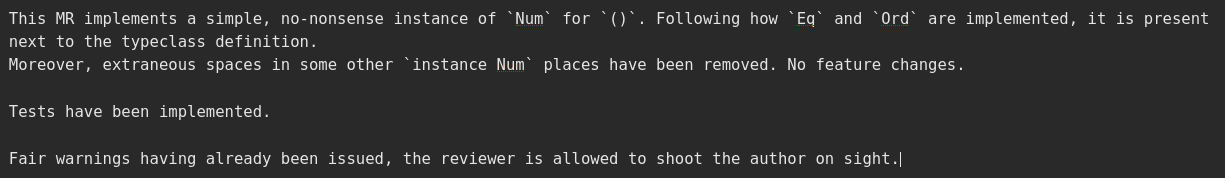It all started with a bad pun.

sadly, unit does not have a `Num` instance

And needless to say that things went downhill from there.

After consulting with famous universally-quantified Sarah @MxLambda and Cale-from-IRC, I was ready to start. But the thing that made me go down this path of despair and shitposting was the gentle push from Romeu Moura, local paradigm jumper and Twitter supermodel, who provided the supply of absolute units needed for this journey.

## “Those are rookie Nums! You gotta pump those Nums up!”

Mathematical theory aside, the `Num` typeclass is the one that indicates that a datatype supports mathematical operations.
This typeclass holds no strong opinion regarding its laws, but I will make a point in respecting them. These laws are:

• Associativity of `(+)`
(x + y) + z = x + (y + z)
• Commutativity of `(+)`
x + y = y + x
• `fromInteger` 0 is the additive identity
x + fromInteger 0 = x
• `negate` gives the additive inverse
x + negate x = fromInteger 0
• Associativity of `(*)`
(x × y) × z = x × (y × z)
• `fromInteger` 1 is the multiplicative identity
x × fromInteger 1 = x and fromInteger 1 × x = x
• Distributivity of `(*)` with respect to `(+)`
a × (b + c) = (a × b) + (a × c) and (b + c) × a = (b × a) + (c × a)

So, nothing very hardcore, these equations are easily translated in Haskell, and that is exactly what I did for the test suite:

``````module Main where

main :: IO ()
main = mapM_ tryTest tests
where
tryTest :: (String, Bool) -> IO ()
tryTest (name, test) = if test then pure () else putStrLn \$ "Could not verify " <> name
tests :: [(String, Bool)]
tests = [ ("Associativity of addition", associativityOfPlus)
, ("Associativity of multiplication", associativityOfTimes)
, ("Distributivity of * with respect to +", distributivity)
]

associativityOfPlus :: Bool
associativityOfPlus = (() + ()) + () == () + (() + ())

associativityOfTimes :: Bool
associativityOfTimes = (() * ()) * () == () * (() * ())

additiveInverse = () + (-()) == fromInteger 1

commutativityOfPlus :: Bool
commutativityOfPlus = () + () == () + ()

multiplicativeIdentity :: Bool
multiplicativeIdentity = first && second
where
first  = () * fromInteger 1 == ()
second = fromInteger 1 * () == ()

distributivity :: Bool
distributivity = first && second
where
first = () * (() + ()) == (() * ()) + (() * ())
second = (() + ()) * () == (() * ()) + (() * ())
``````

With this rigorous test suite, we can now proceed to the implementation of a law-abiding `Num` instance for `()`. Lo and behold.

``````instance Num () where
() + ()        = ()
() * ()        = ()
() - ()        = ()
abs ()         = ()
fromInteger _n = ()
signum _n      = ()
``````

And the tests pass.

## Getting it into GHC

In light of this success, the idea of getting this instance merged into GHC finally stuck in my head and a merge request was submitted. In all fairness, I didn’t expect that it would be merged, but I was interested in the technicalities of “why”.Which immediatly prompted a very interesting remark from Oleg Grenrus, aka. Phadej, about the defaulting behaviour in GHCi. This feature enables the REPL to automatically specialise a number litteral, say 54, to a type that was designed as “making sense” through defaulting rules. The point is that when a Haskell beginner enters “1” in the REPL, they should get `it :: Int`, not `Num a => a`, which is a tad scary when one does not have any notion of typeclasses, and especially not familiar with Haskell’s numerical tower. Kwang Yul Seo’s article about type defaulting behaviour (2017) summarises the following rules:

``````default Num Integer
default Real Integer
default Enum Integer
default Integral Integer
default Fractional Double
default RealFrac Double
default Floating Double
default RealFloat Double
``````

And a quick test in the REPL indicates that `Num ()` seems to be picked at the default specialisation for numbers. Which is pretty bad.

RIP 💀.

## Conclusion

This was a fun ride! I was far from imagining that this kind of bad puns would lead me 6 hours later to a merge request in GHC, but I think the most important part is that I got to learn about the tricky behaviour of defaulting in GHCi.
I also wish to thank Oleg for his patience in reviewing my merge request.

But also that was very fun.
Cheers!